# How to get the Address of Active Cell in Excel

Address of cell in excel generally refers to the location of some cell. Active cell can be defined as the cell that contains some data or can be active due to some user click or any other associated activities. Getting the active cell address can be important to understand the point of processing. It became more important when user is dealing with large spreadsheet. For this tutorial let’s try to evaluate two ways to determine the address of active cell. First process is based on excel user defined formula, and second process is based on the “VBA” code.

List of solutions that allow user to evaluate the address of an active cell are given below:

• First way is to use the formula.

• Another way is to use the excel visual basic analysis code.

Let’s understand the first way to solve the problem with an example by using the formula method.

## Examples to Obtain the Address of Active Cells in Excel

Step 1

Before, starting the example user need to create an excel workbook. Normally create a workbook and store it on any valid location within your computer. To understand the changes and effects clearly, let’s assume the below given spreadsheet.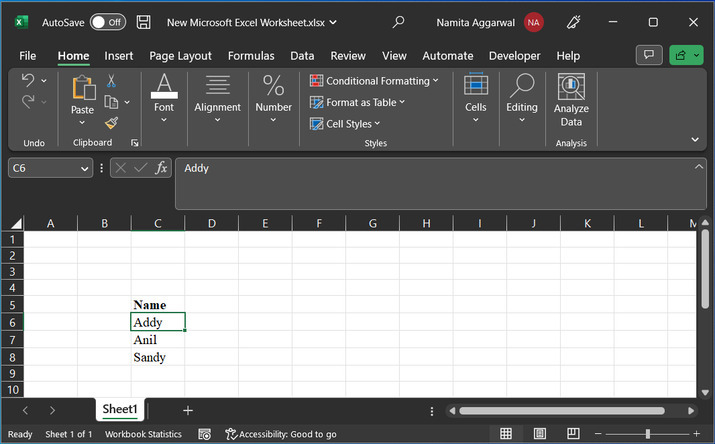From the above spreadsheet the column C and row 6 seems to be active, that is the cell C6 is an active cell. Now, let’s understand the formula used to evaluate this address directly without any need to obtain the address manually.

Step 2

The formula to access the address of active cell is given below:

The above provided formula uses address method. This method takes two input parameters such as row() and column(). As, the address of excel active cell is a combination of both row and column.

Now, let’s understand, how to apply this formula on the excel worksheet.

Simply go to the active cell and type the formula “=ADDRESS(ROW(), COLUMN())”. Consider below provided snapshot for reference: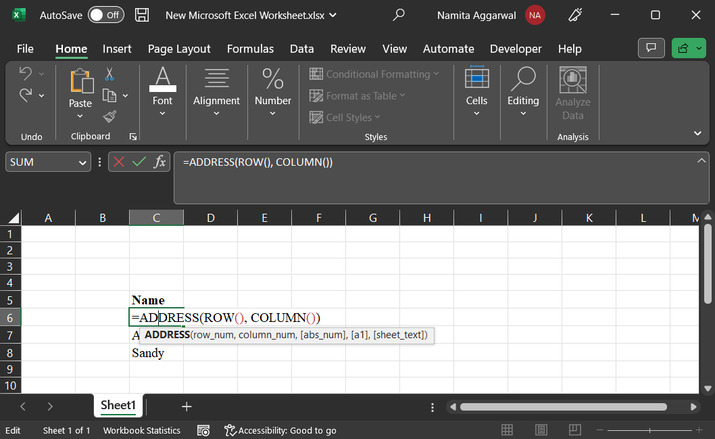Step 3

After typing the above provided formula, simply press “Enter” key. This will display the sheet address inside the active cell. Consider below given image for active cell: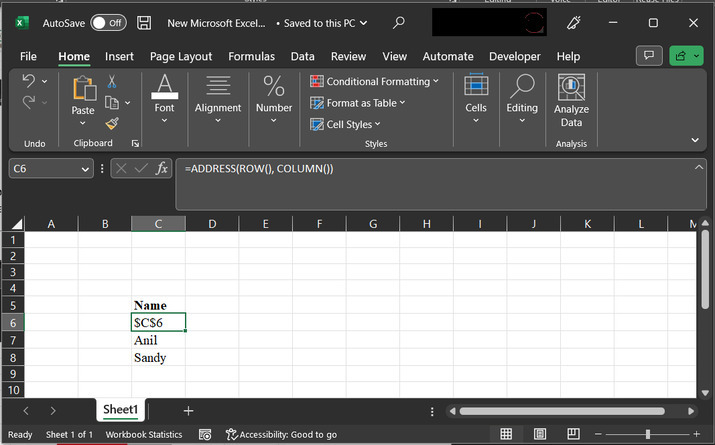Hence, for this example the address obtained for active cell is C6.

## Example 2

Step 1

Now, let’s consider another example, this sample worksheet contains data for the employee ID. This example will help student to understand the way of using the Excel VBA code to evaluate the address of active cell.

Consider the below given excel worksheet, with active C8 cell.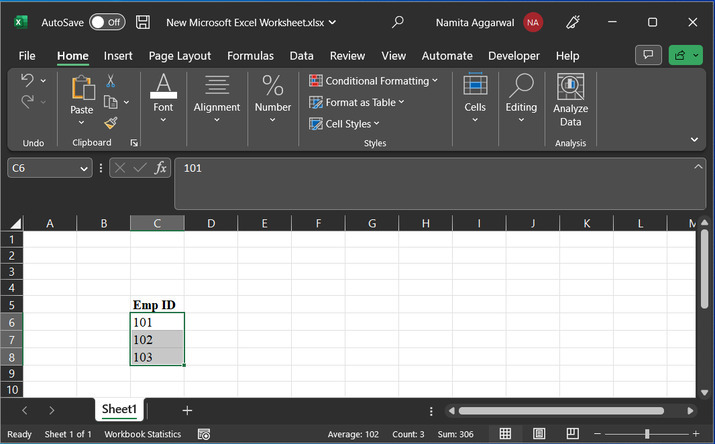Step 2

Before writing the code, student need to open the code editor. To open the code area, go to the “Developer” tab, then select “Visual code” from the code section.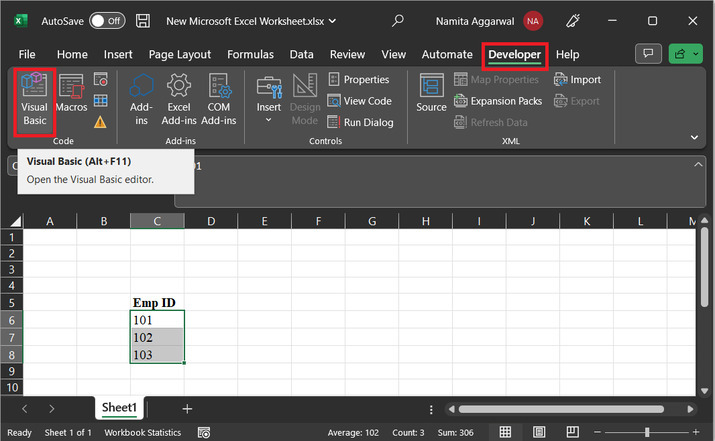Step 3

This will open the Microsoft visual basic for applications, as depicted below: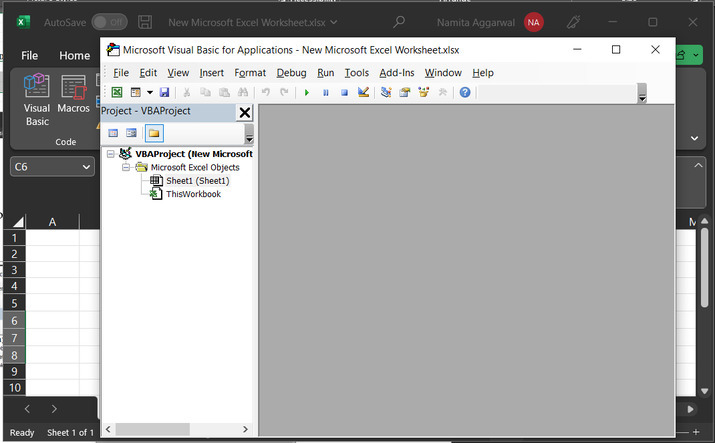Step 4

To write the module definition, student need to create a module. To create a new module, go to insert then click on module.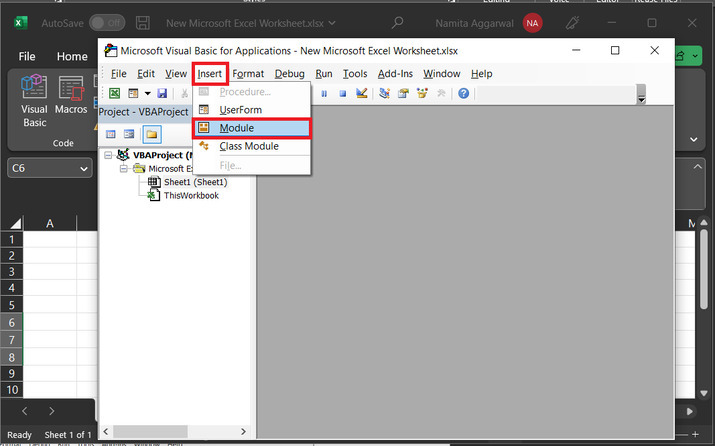Step 5

This will open the below given code editor: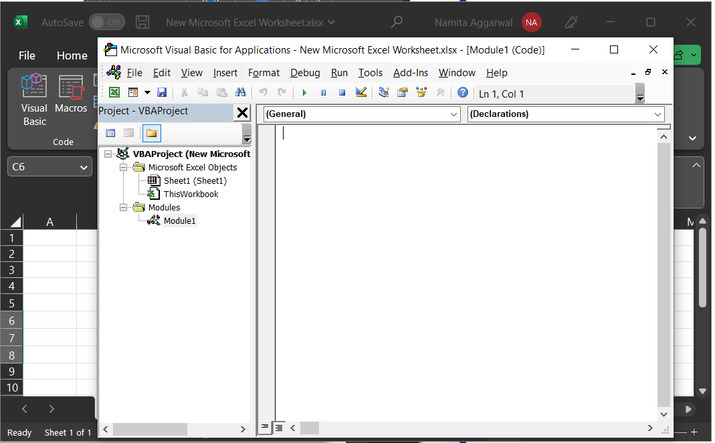Step 6

Type below given code to code area:

' define method for active cell
Sub Example_of_Active_Cell()
' calling message box with activecell
' end of module
End Sub


### Snapshot for code area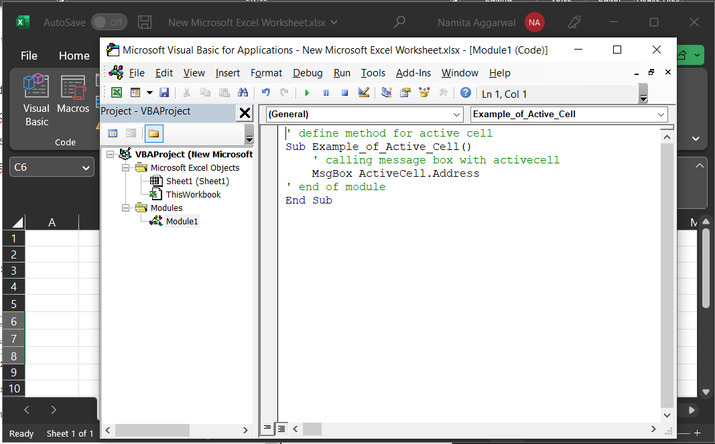Click on “Run”, shown below: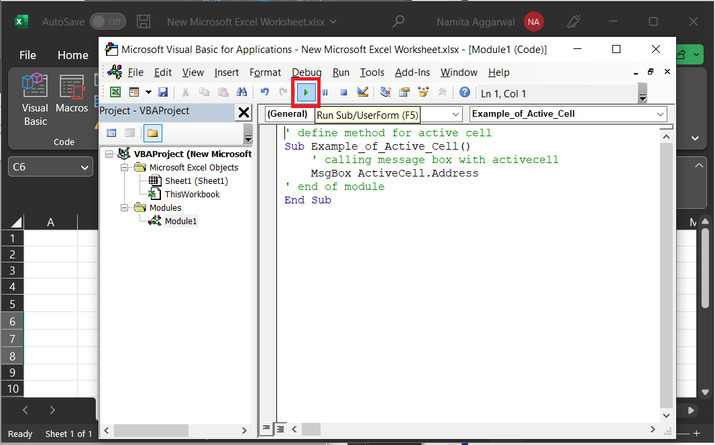Step 7

This will open the “Macros” dialog box. Click again on “Run”.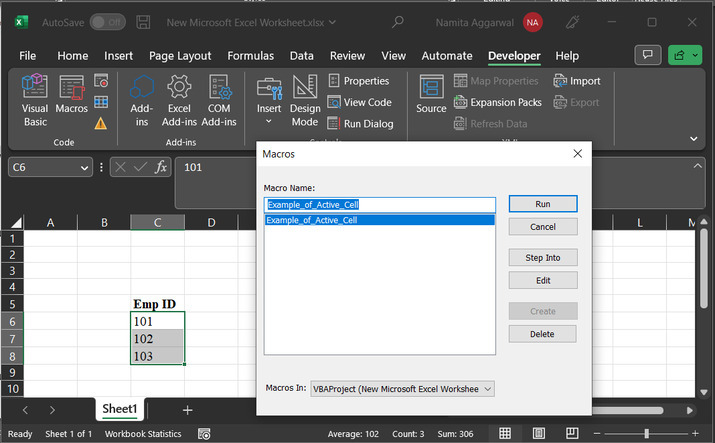Step 8

This will display the required results: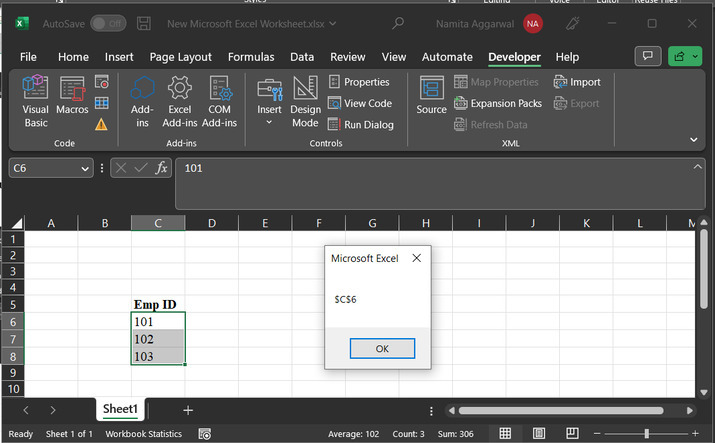Click “OK”, to remove the appeared result from the screen.

## Conclusion

In this tutorial, two examples are used to demonstrate “how user can obtain can address of active cell”. First method is to use formula by using the address() method. On the other hand, second method, illustrate the way to use VBA code, to perform the same task. Both the methods are efficient and can work according to the user requirement.

Updated on: 22-Aug-2023

121 Views